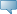## Detailed Course Information

Fall 2019
Oct 26, 2021Select the desired Level or Schedule Type to find available classes for the course.

 ENG 1450 - Introduction to Electrical and Computer Engineering (Lab required) Part I; Current, voltage, energy, potential, power Ohm's law; independent sources; capacitor, inductor, ideal diode, op-amp; Kirchoff's law; simple circuits (Resistive, RC, RL, OP-Amp; Diode); introduction to ac theory (Sinusoidal waveform, phase relations of voltage and current waveforms for R,L,C. RL and RC circuits). Part II; Applications (Digital Logic, motors). Prerequisites:[A minimum grade of 60% in Pre-Calculus Mathematics 40S (or a minimum grade of “C” in one of MATH 0401, MATH 1230, MATH 1500, MATH 1501, MATH 1510, MATH 1520, MATH 1690, MSKL 0100 or the former MATH 1680)] and [a minimum grade of 60% in Physics 40S (or passing grade in PHYS 0900 or PSKL 0100; or a minimum grade of “C” in PHYS 1050 or PHYS 1051)] and [a minimum grade of 60% in Chemistry 40S (or passing grade in CHEM 0900 or CKSL 0100; or minimum grade of “C” in CHEM 1300 or CHEM 1301)] or their equivalents. - 3.0 Credit hours Engineering, Engineering lab, RO admin use only Engineering Prelim Year Department Course Attributes: Science Requirement for BA, Recommended Intro Courses